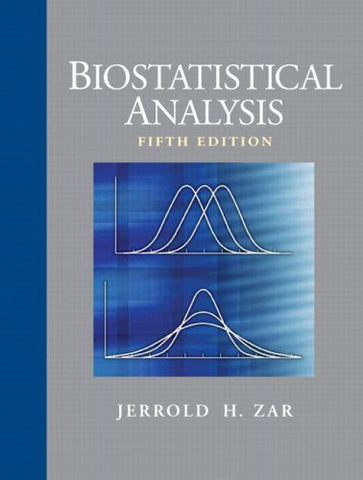# Biostatistical Analysis (5th Edition)

• \$409.84
• Save \$29
• Binding: Hardcover
• Author: Jerrold H. Zar
• Publish Date: 2009-02-25

Attention : For textbook, access codes and supplements are not guaranteed with used items.

Zarâs Biostatistical Analysis, Fifth Edition, is the ideal book for readers seeking practical coverage of statistical analysis methods used by researchers to collect, summarize, analyze and draw conclusions from biological research. The latest edition of this best-selling textbook is both comprehensive and easy to read. It is suitable as an introduction for beginners and as a comprehensive reference book for biological researchers and other advanced users.

Â

Introduction; Populations and Samples; Measures of Central Tendency; Measures of Dispersion and Variability; Probabilities; The Normal Distribution; One-Sample Hypotheses; Two-Sample Hypotheses; Paired-Sample Hypotheses; Multisample Hypotheses: The Analysis of Variance; Multiple Comparisons; Two-Factor Analysis of Variance; Data Transformations; Multiway Factorial Analysis of Variance; Nested (Hierarchical) Analysis of Variance; Multivariate Analysis of Variance; Simple Linear Regression; Comparing Simple Linear Regression Equations; Simple Linear Correlation; Multiple Regression and Correlation; Polynomial Regression; Testing for Goodness of Fit; Contingency Tables; More on Dichotomous Variables; Testing for Randomness; Circular Distributions: Descriptive Statistics; Circular Distributions: Hypothesis Testing

Â

For all readers interested in biostatistics.